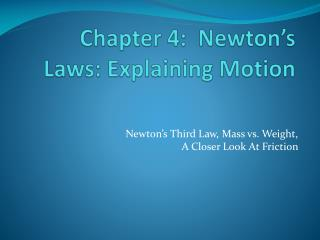Download PresentationChapter 4: Newton’s Laws: Explaining Motion

# Chapter 4: Newton’s Laws: Explaining Motion - PowerPoint PPT Presentation

Chapter 4: Newton’s Laws: Explaining Motion. Newton’s Third Law, Mass vs. Weight, A Closer Look At Friction. Newton’s Third Law. To every action there is an equal but opposite reaction.I am the owner, or an agent authorized to act on behalf of the owner, of the copyrighted work described.
Download Presentation## Chapter 4: Newton’s Laws: Explaining Motion

Download Policy: Content on the Website is provided to you AS IS for your information and personal use and may not be sold / licensed / shared on other websites without getting consent from its author.While downloading, if for some reason you are not able to download a presentation, the publisher may have deleted the file from their server.

- - - - - - - - - - - - - - - - - - - - - - - - - - E N D - - - - - - - - - - - - - - - - - - - - - - - - - -
Presentation Transcript
1. Chapter 4: Newton’s Laws: Explaining Motion Newton’s Third Law, Mass vs. Weight, A Closer Look At Friction

2. Newton’s Third Law • To every action there is an equal but opposite reaction. • To every action there is always opposed an equal reaction: or the mutual actions of two bodies upon each other are always equal, and directed to contrary parts. • Every force occurs as one member of an action/reaction pair of forces. • The two members of an action/reaction pair act on two different objects. • The two members of an action/reaction pair are equal in magnitude but opposite in direction: FAon B = -FBon A

3. Newton’s Third Law Continued • To make a complete diagram of all forces involved, you must include all objects involved in the interaction • A FBD only takes one object and labels all external forces acting on that one object

4. Example 1A book resting on a table:

5. Example 2A ball swinging on a string:

6. Mass vs. Weight • Mass and weight are not the same thing, but they are related • Mass, m • An object’s mass is a measure of the amount of matter the object contains. • An intrinsic property of an object. • How much an object resists a change in its motion. • The greater the mass the greater the inertia or resistance to change • The greater the mass the smaller the acceleration provided by a given force.

7. Fg = W Mass vs. Weight Continued • Weight, Fg • w = mg (downward, toward the center of the agent) • It is directly proportional to mass, but not equal to it. • Weight is not a fixed quantity, it is dependent on the location of the object. • The Weight of an object on the Earth is not the same as the Weight of an object on the moon.

8. Apparent Weight • Weight is the force of gravity on an object • You cannot feel it directly • Your sensation of weight, or how heavy you feel, is due to contact forces • If you stand at rest, a = 0, the force you feel is the normal force pressing on you, which is exactly equal in magnitude to your weight. • If a ≠ 0, you will not “feel” your weight, but rather you will feel what is called your “apparent” weight or the magnitude of the contact force that supports you (the normal force)

9. Apparent Weight ContinuedExample: An elevator standing still vs. moving upward y y a wapp wapp x x w =Fg w =Fg a = 0 wapp - Fg = ma wapp - mg = ma wapp = mg + ma wapp = mg(1 + a/g) wapp = w(1 + a/g) wapp - Fg = ma = 0 wapp = Fg wapp = w

10. Apparent Weight Continued • As the elevator goes upward, you feel heavier because a > 0, so wapp > w • As the elevator goes downward, you feel lighter because a < 0, so wapp < w

11. Question1: Would a bathroom scale read the same thing on Earth and on the moon?

12. Question 2: A car with a weight of 15,000N is being towed up a 20° slope at constant velocity. Friction is negligible. The tow rope is rated at 6000N maximum tension. Will it break?

13. Friction • Static friction changes depending on how much force is applied to the object. • An object remains at rest as long as fs < fsmax • The object slips when fs = fsmax • Once the object starts to move after attaining its maximum static frictional force, it now has kinetic friction acting on it. • Kinetic friction, unlike static friction, has a nearly constant magnitude and is always less than that of the maximum static friction force. • It is much harder to start an object moving than it is to keep the object moving.